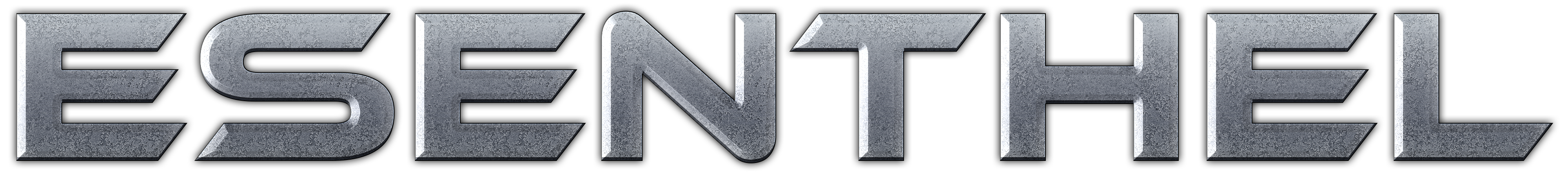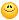About Store Forum Documentation ContactRotating and Moving the Player Obj in depth Author Message
ZapaMember

 Post: #1 Rotating and Moving the Player Obj in depth Hi, My name is Constantin, a 20 year old Game Designer (currently working in the field for a Mobile Game Dev company), who happened to run into Esenthel not long ago! In the past I've been using another SDK (DarkGDK) for my game dev experiments (been coding for 5 years actually), but I never got passed to using any other SDK/Engine, so I'm kinda stuck with coding the GDK Way. Anyway, I picked up some bad coding habits in the past, that still hunt me to this day, and I'm running into some problems when it comes to working with Esenthel. Recently, I played with the idea of working on a 3D Side Scrolling, story driven game. I read some of the Tutorials that come with Esenthel, and tried to adapt the Character tutorial to my needs. I've got the camera to follow the player's position, while keeping it stuck on a 90' angle, and changed the movement code to allow moving left/right with D and A, but I'm stuck at moving the player on the Z axis (moving the player in depth). What I want to do is the following: [pseudo code] if W_Key is pressed then rotate the player 90' move player on that direction end; [/pseudo] But if I temper with with input.turn.x/y and then press the W, the Player Actor kind moves in circles. Here's an example of my code: Code: ```       if(Kb.b(KB_D))  //Move forward with D        {            input.move.z = 1;        }        else if(Kb.b(KB_A))  //Move backwrd with S        {            input.move.z = -1;        }        else        {            input.move.z = 0;        }        if(Kb.b(KB_W)) // Move in Depth with W        {                       input.move.x = -1;                   }        else if (Kb.b(KB_S)) // Move in -Depth with S        {            input.move.x = 1;        }        else        {            input.move.x = 0;        }``` Anyway, I guess that I'm used to the old way of turning and moving an object, and I'm trying to do the following: [pseudocode] if W_Key is pressed RotateObject(90') myObject.SetPositionZ(myObject.GetPositionZ()+1) end; [/end of pseudocode] Thing is, I'm trying to rotate the object, and reset it's position to the direction it is facing. Sorry if my way of explaining it is a little wierd. If it's not that understandable, I will make a small example using GDK on what I want to accomplish. Thank you for your time, Zapa
08-27-2011 12:13 PM
ZervoxMemberPost: #2 RE: Rotating and Moving the Player Obj in depth //Rotates left with A and Right with D using the input angles, meaning the player will have smooth turning and kind of leaning effekt Code: `input.turn.x=Kb.b(KB_A)-Kb.b(KB_D);` //Left with A and Right with D Code: `input.move.x=Kb.b(KB_D)-Kb.b(KB_A);` Forward with W and backwards with S Code: `input.move.z=Kb.b(KB_W)-Kb.b(KB_S);` if you just want rotation Rotate 90 degrees left when KB_A is pressed Code: `if(Kb.bp(KB_D))LocalPlayer.angle.x-=DegToRad(90);` Rotate 90 degrees right when KB_D is pressed Code: `if(Kb.bp(KB_A))LocalPlayer.angle.x+=DegToRad(90);` (This post was last modified: 08-27-2011 12:42 PM by Zervox.)
08-27-2011 12:38 PM
ZapaMember

 Post: #3 RE: Rotating and Moving the Player Obj in depth Hi Zervox, Thanks for your answer, but this is the default movement behavior that I want to avoid. When I press W_Key, I want the player to face "North" and move in that direction, not rotate. So just face north, move in that direction. Then, going from W to D, he should fact West and move in that direction. I have attached an image to further explain in more detail what I meant.A better example would be gameplay footage from BountyArms: http://www.youtube.com/watch?v=ecD9OtYN8uE 0:27 is when he moves closer to the "camera" after going Left/Right. Also, didn't know there was a DegToRad Converter, thanks for that! Started writing my own a few minutes ago08-27-2011 01:40 PM
ZervoxMemberPost: #4 RE: Rotating and Moving the Player Obj in depth I guess like this you mean? Code: ```if(Kb.b(KB_W)) {     input.move.z+=1;     angle.x = DegToRad(0); } if(Kb.b(KB_S)) {     input.move.z+=1;     angle.x = DegToRad(180); } if(Kb.b(KB_D)) {     input.move.z+=1;     angle.x = DegToRad(270); } if(Kb.b(KB_A)) {     input.move.z+=1;     angle.x = DegToRad(90); }``` (This post was last modified: 08-27-2011 02:06 PM by Zervox.)
08-27-2011 02:06 PM
ZapaMember

 Post: #5 RE: Rotating and Moving the Player Obj in depth Thanks Zervox, this was what I was aiming for! All the best.
08-27-2011 02:19 PM
ZervoxMemberPost: #6 RE: Rotating and Moving the Player Obj in depth No problem had the code lying around anyways, but I now I wanted to finish it with same controls as on your link. Code: ```if(Kb.b(KB_W) && !Kb.b(KB_A) && !Kb.b(KB_D) && !Kb.b(KB_S)) {     input.move.z+=1;     angle.x = DegToRad(0); }                                  if(Kb.b(KB_W) && Kb.b(KB_A) && !Kb.b(KB_D) && !Kb.b(KB_S)) {     input.move.z+=1;     angle.x = DegToRad(45); } if(Kb.b(KB_W) && Kb.b(KB_D) && !Kb.b(KB_A) && !Kb.b(KB_S)) {     input.move.z+=1;     angle.x = DegToRad(315); } if(Kb.b(KB_S) && !Kb.b(KB_D) && !Kb.b(KB_A) && !Kb.b(KB_W)) {     input.move.z+=1;     angle.x = DegToRad(180); } if(Kb.b(KB_S) && Kb.b(KB_A) && !Kb.b(KB_D) && !Kb.b(KB_W)) {     input.move.z+=1;     angle.x = DegToRad(135); } if(Kb.b(KB_D) && Kb.b(KB_S) && !Kb.b(KB_A) && !Kb.b(KB_W)) {     input.move.z+=1;     angle.x = DegToRad(225); } if(Kb.b(KB_D)&& !Kb.b(KB_S) && !Kb.b(KB_W) && !Kb.b(KB_A)) {     input.move.z+=1;     angle.x = DegToRad(270); }                  if(Kb.b(KB_A)&& !Kb.b(KB_S) && !Kb.b(KB_D)&& !Kb.b(KB_W)) {     input.move.z+=1;     angle.x = DegToRad(90); } if(!Kb.b(KB_W) && !Kb.b(KB_S) && !Kb.b(KB_A) && !Kb.b(KB_D)) {     input.move.z=0; }```
08-27-2011 02:31 PM
 « Next Oldest | Next Newest »